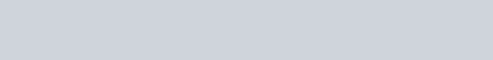# Diabetes Prediction using Decision Tree in Python

Hello Everyone,

In this tutorial, we are going to build a prediction model using the decision tree in Python using the scikit-learn machine learning module. Let’s first understand what is a decision tree.

## What is a Decision Tree?

Decision Tree is one of the popular classification and prediction algorithms. A decision tree is a tree-like structure that kind of looks like a flowchart. In a decision tree, each internal node denotes a test on an attribute, each branch represents an outcome of the test, and each leaf node holds a class label.

Decision Tree is a supervised learning algorithm. That is a type of machine learning in which machines are trained using well “labeled” training data and on basis of that data, machines predict the output. The labeled data implies that some input data is already tagged with the correct output.

Now, let’s build a prediction model for diabetes detection. For that, we need to first need to download the dataset for diabetes. You can download it from the below link:

https://www.kaggle.com/datasets/uciml/pima-indians-diabetes-database

In this dataset we will deal with 8  attributes of data and ‘Outcome’ will be our labeled column. Now, let’s see how we are going to build our prediction model for checking whether a person is diabetic or not.

## Code for Diabetes Prediction

For building this prediction model we need Python libraries like NumPy. Pandas and sklearn. So if you don’t have the libraries you can install them on your machine using the following commands.

`pip install numpy`
```pip install pandas
pip install -U scikit-learn```

After installing all the libraries, we need to import these mentioned below: NumPy, pandas, and scikit-learn. Using sklearn we will import modules train_test_split which will split our data into training and testing datasets and using accurracy_score we can check the accuracy of our model

```import numpy as np
import pandas as pd
from sklearn.preprocessing import StandardScaler
from sklearn.model_selection import train_test_split
from sklearn.tree import DecisionTreeClassifier
from sklearn.metrics import accuracy_score```

Now using the Pandas library we will load our CSV file using the command below. Using the `head()` function we can see the first five values of the dataset. So we can see the attributes we will consider for prediction: Pregrancies, Glucose, Blood Pressure, Skin Thickness, BMI(Body Mass Index), Age, Insulin, diabetes pedigree function, and Outcome(0:Non-diabetic,1:diabetic).

```diabetes_dataset=pd.read_csv('diabetes.csv')

Output:Now, let’s just check how many values we have in our dataset using the .shape function which will give us a number of rows and columns in our dataset. For our dataset no.of rows are 765 and 9 columns.

`diabetes_dataset.shape`

Output:

`(765, 9)`

Now let’s see the description of the dataset, which will give a 5-quartile number summary and mean and standard deviation. A summary consists of five values: the most extreme values in the data set (the maximum and minimum values), the lower and upper quartiles, and the median.

`diabetes_dataset.describe()`

Output:Now we have to concentrate on the outcome column which is a label for prediction because it will give us the answer to whether the person has diabetes or not. So we will now separate label and data. For this data, the label will be the ‘Outcome’ column.

`diabetes_dataset['Outcome'].value_counts()`

Output:

``
```#0->non-diabetic 1->diabetic
diabetes_dataset.groupby('Outcome').mean()```

Output:Now we will save the data without the labeled column in the variable X  using drop() function and the labeled column in the Y. Now let’s just check using print() what we get in both X and Y.

```#seperating data and label
X=diabetes_dataset.drop(columns='Outcome',axis=1)
Y=diabetes_dataset['Outcome']
print(X)
```

Output:`print(Y)`

Output:Now to train our model we first need to normalize our data as we have many different values for each attribute and it can become difficult for a model to train. So we will standardize the data using StandardScaler() function. So will standardize the data in X.

```#data standardization
scaler= StandardScaler()
scaler.fit(X)
standardized_data=scaler.transform(X)
X=standardized_data
print(X)

```

Output:Now for checking purposes, we will split our data using train_test_split() we will split our data into an 80-20 ratio. So, 80% of the data will be our training data and 20% will be our testing data.

```#data test and train
X_train,X_test,Y_train,Y_test=train_test_split(X,Y,test_size=0.2,stratify=Y,random_state=2)
print(X.shape,X_train.shape,X_test.shape)```

Output:

`(765, 8) (612, 8) (153, 8)`

Now for the classification of the data, we are using a decision tree. Now let’s assign the decision tree classifier to the classifier variable and let’s fit our training data to the classifier which we have stored in X_train and Y_train. And after that let’s make a prediction using predict() on the classifier.

```classifier=DecisionTreeClassifier()
classifier.fit(X_train,Y_train)
#model evaluation
X_train_prediction=classifier.predict(X_train)
training_data_accuracy=accuracy_score(X_train_prediction,Y_train)```

Now that we have to build a prediction model, let’s check the accuracy of our training data as accuracy for any prediction model to be reliable.

`print(training_data_accuracy)`

Output:

`1.0`

Now let’s assign testing data to our classifier which we have stored in X_train with the predict() function and let’s check the accuracy for testing data also. As testing data will help us knowing actually how our prediction model is performing.

```X_test_prediction=classifier.predict(X_test)
testing_data_accuracy=accuracy_score(X_test_prediction,Y_test)
#decision tree
print(testing_data_accuracy1)```

Output:

`0.99`

Now let’s give a tuple of values in the sequence of data that was in our available dataset and using NumPy we will change the input data into a NumPy array. And then will reshape the data and assign it to the classifier and let’s check the prediction of the given values whether the given person is diabetic or not.

```input_data=(9,170,74,31,0,44,0.403,43)
#changing input data to numpy
input_data_numpy=np.asarray(input_data)
#reshape the array
input_data_reshape=input_data_numpy.reshape(1,-1)
#standar input data
std_data=scaler.transform(input_data_reshape)
#print(std_data)
prediction=classifier.predict(std_data)
print(prediction)

if prediction==0:
print("The person is non-diabetic")
else:
print("Person is diabetic")```

Output:So we have successfully built a prediction model using a decision tree